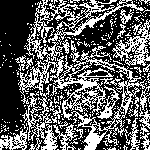# Chris Basmajian / Work / False Binaries (Black & White)

False Binaries (Black & White) (Series)

2008
Interactive video (video camera, display, custom software, computer)

The works in this series capture live video of the viewer, and translate each grayscale pixel's binary representation (electronic data) into a black or white representation (visual) to create a binary (1-bit) image. These arbitrary translations introduce the difference between the two forms of representation as noise. Compared to a typical 1-bit threshold image, this noise can increase the image's perceptual resolution.

Demos: The downloadable demos below are Java applications created with Processing. A Mac with a good web camera (iSight recommended) is required to run them. Place the camera in a stationary position and in a well lit areaCycling Sample Index

For each new video frame a sample index increments, cycling from 0 to 7. The bit at the sample index of each 8-bit source pixel is displayed as a 1-bit color: black for 0 or white for 1.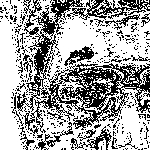Bit Sum Threshold

For each 8-bit source pixel, the bits are summed. If the sum of the 8 bits is greater than or equal to 4, the pixel is displayed as white. If the sum is less than 4, the pixel is displayed as black.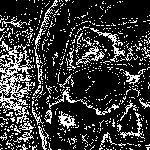Cycling Bit Sum Threshold

For each new video frame a threshold number increments, cycling from 0 to 7. For each 8-bit source pixel, the bits are summed. If the sum of the 8 bits is greater than or equal to the threshold, the pixel is displayed as white. If the sum is less than the threshold, the pixel is displayed as black.Cycling Bit Sum Match

For each new video frame a match number increments, cycling from 0 to 7. For each 8-bit source pixel, the bits are summed. If the sum of the 8 bits equals the match number, the pixel is displayed as white. If the sum does not equal the match number, the pixel is displayed as black.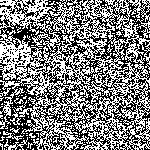Bit Sum Odd/Even

For each 8-bit source pixel, the bits are summed. If the sum of the 8 bits is an odd number, the pixel is displayed as white. If the sum is an even number, the pixel is displayed as black.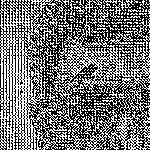Average Color Bit Grid

Each new video frame is divided into 3 pixel x 3 pixel grids. The 9 pixels in each grid are averaged together into a single 8-bit value. Each bit in this value is assigned to a pixel in the grid. Each pixel is displayed as white if its corresponding bit is 1 and black if its bit is 0. The pixel at the center of each grid is displayed as black.